# Quant Quiz for IBPS CLERK MAINS 2018 | 9th January 2019

Quant Quiz to improve your Quantitative Aptitude & Data Interpretation. For upcoming exams like Bank, SSC, Railway, UPSC, UPSSSC, CDS, UPTET, KVS, DSSSB and other Government exams.

Q1. A laborer was appointed by a contractor on the condition he would be paid Rs 75 for each day of his work but would be, fined the rate of Rs 15 per day for his absent. After 20 days, the contractor paid the laborer Rs 1140. The number of days the laborer absented from work days:

(a) 3 days

(b) 5 days

(c) 4 days

(d) 2 days

(e) None of these

Q2. 9 kg of rice costs as much as 4 kg of sugar, 14 kg of sugar costs as much as 1.5 kg of tea, 2 kg of tea costs as much as 5 kg of coffee. Find the cost of 2.5 kg of rice, if 11 kg of coffee costs Rs. 462.

(a) Rs. 13.50

(b) Rs. 14

(c) Rs. 15

(d) Rs. 12.50

(e) none of these

Q3. A box contains 30 eggs out of which 6 are rotten. Two eggs are chosen at random. What is the probability that at least one of the chosen eggs is rotten ?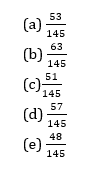Q4. In an assembly election, a candidate got 55% of the total valid votes. 2% of the total were declared invalid. If the total number of voters is 104000, then the number of valid votes polled in favor of the candidate is.

(a) 56506

(b) 56650

(c) 56560

(d) 56056

(e) None of these

Q5.  3 years ago, Ambuj was thrice as old as Avinash. If he ratio of their present ages is 8 : 3 respectively, what is the difference between their present ages in years ?

(a) 32 years

(b) 30 years

(c) 28 years

(d) 35 years

(e) None of these

Directions (Q6-Q10): In each of these questions, two equations (I) and (II) are given. You must solve both the equations and give answer

(a) if x < y

(b) if x ≤ y

(c) if x = y or no relation can be established

(d) if x > y

(e) if x ≥ y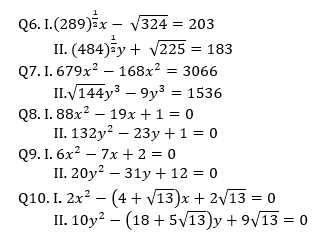# Solutions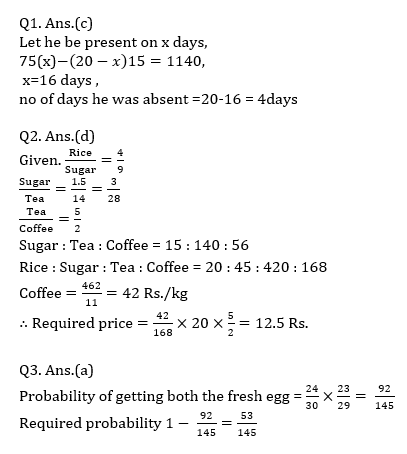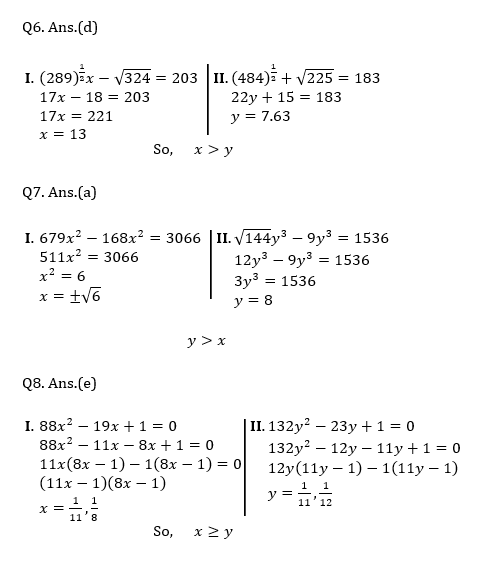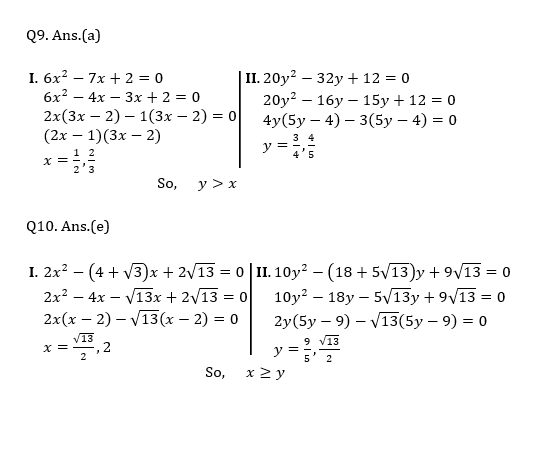## WhatsApp Group Join here

Mail us at : ambitiousbaba1@gmail.com